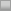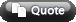View Single Post01-20-2008, 03:09 AM #2 schubes journeyman   Join Date: Jul 2004 Posts: 229 Re: Maximizing EV to Find a GTO Betsize I can't make any sense of your equation or definition of a, but I'll show you how to find the optimal bet size (ignoring the semibluff aspect for now). For simplicity I'll say p=1, we can always change the scale of our answers, so there's no loss of generality. Villain wants to call enough to make Hero indifferent to bluffing and checking his bluffing hands. If you solve the indifference equation you'll find he'll call with 1/(s+1) of hands, including his nut hands. So hero gets no EV from his bluffing hands and his (ex-showdown) EV for his value bets is: EV = n*-s + (1/(s+1) - n)*s = s/(s+1) -2ns To find the max EV we set the derivative equal to zero... dEV/ds = 1/(s+1) - s/(s+1)^2 -2n = 0 --> (s+1)^2 - 1/2n = 0 So the optimal bet size is s = -1 + (2n)^-.5 For n >= 1/2, the optimal bet size is zero as we'd expect if n=2/9, it's half the pot if n=1/8, it's pot size etc.